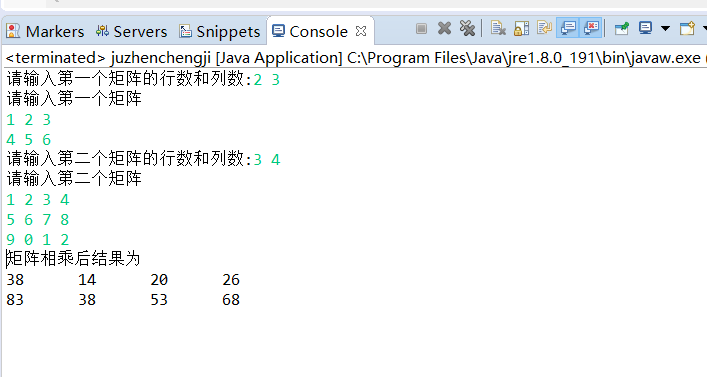• 三角矩阵分上三角矩阵和下三角矩阵两种。上三角矩阵的对角线左下方的系数全部为零，下三角矩阵的对角线右上方的系数全部为零。三角矩阵可以看做是一般方阵的一种简化情形。比如，由于带三角矩阵矩阵...

详细的代码可见github：
https://github.com/AbitGo/myClassWork/tree/master/workspace_ds
三角矩阵是方形矩阵的一种，因其非零系数的排列呈三角形状而得名。三角矩阵分上三角矩阵和下三角矩阵两种。上三角矩阵的对角线左下方的系数全部为零，下三角矩阵的对角线右上方的系数全部为零。三角矩阵可以看做是一般方阵的一种简化情形。比如，由于带三角矩阵的矩阵方程容易求解，在解多元线性方程组时，总是将其系数矩阵通过初等变换化为三角矩阵来求解；又如三角矩阵的行列式就是其对角线上元素的乘积，很容易计算。有鉴于此，在数值分析等分支中三角矩阵十分重要。一个所有顺序主子式不为零的可逆矩阵A可以通过LU分解变成一个单位下三角矩阵L与一个上三角矩阵U的乘积。
具体实现类：
package com.company.ch5;
public class HuffmanTree {
public int[][] huffmanfiCoding(int[] w){
//字符个数
int n = w.length;
//结点数
int m = 2*n -1;
HuffmanNode[] HN = new HuffmanNode[m];
int i;
for(i = 0;i
测试类：
package com.company.ch4.Matrix;
public class TriangularMatrixTest {
public static void main(String[] args) {
System.out.println("上三角矩形");
TriangularMatrix triangularMatrix1 = new TriangularMatrix(8,true,99);
triangularMatrix1.autoGenerate();
triangularMatrix1.disPlay();
System.out.println("下三角矩形");
TriangularMatrix triangularMatrix2 = new TriangularMatrix(8,false,88);
triangularMatrix2.autoGenerate();
triangularMatrix2.disPlay();
}
}
测试结果：
上三角矩形
991223434
9999234345
99999934564
999999994567
9999999999567
99999999999967
999999999999997
9999999999999999
下三角矩形
088888888888888
12888888888888
2348888888888
345688888888
45678888888
56789108888
678910111288
7891011121314

展开全文• 主要介绍了Java数据结构之稀疏矩阵定义与用法,结合实例形式分析了java稀疏矩阵定义、运算、转换等相关操作技巧,需要的朋友可以参考下
• 1.定义两个矩阵数组，先判断矩阵a与b是否可以相乘 2.若不可以，输出矩阵不能相乘，若可以，定义乘积后的矩阵数组为c，使c=a*b，遍历输出c 3.其中定义c为 c[ a的行数 ][ b的列数 ],遍历a,b使他们乘积 主函数 1.编写...
一、思路 函数 1.定义两个矩阵数组，先判断矩阵a与b是否可以相乘 2.若不可以，输出矩阵不能相乘，若可以，定义乘积后的矩阵数组为c，使c=a*b，遍历输出c 3.其中定义c为 c[ a的行数 ][ b的列数 ],遍历a,b使他们乘积 主函数 1.编写输入语句 2.分别输入a与b的行数与列数，构造数组a,b，再输入矩阵a,b 3.调用函数
二、Java程序展示
import java.util.Scanner;
public class juzhenchengji {

public static void chengji(int a[][],int b[][]) {

if(a.length != b.length){                    //判断a的列数与b的行数是否相等
System.out.println("矩阵无法相乘，数组不存在");
}
else {
int x=a.length;
int y=b.length;
int c[][]=new int[x][y];                  //定义乘积后的数组c[ a的行数 ][ b的列数 ]
for(int i=0;i<x;i++)
{
for(int j=0;j<y;j++)
{
for (int k = 0; k < b.length; k++)
c[i][j] += a[i][k] * b[k][j];     //即c[i][j] = a*b + a*b + a*b + ......
}
}
System.out.println("矩阵相乘后结果为");
for(int m = 0;m<a.length;m++)
{
for(int n = 0;n<b.length;n++)
{
System.out.print(c[m][n]+"\t");        //"\t"意思是补空格到当前字符串长度到8的整数倍
}
System.out.println();
}
}
}
public static void main(String[] args) {
Scanner sc = new Scanner(System.in);
System.out.print("请输入第一个矩阵的行数和列数:");
int x1 = sc.nextInt();
int y1 = sc.nextInt();
int[][] arr1 = new int[x1][y1];
System.out.println("请输入第一个矩阵");
for (int i = 0; i < x1; i++) {
for (int j = 0; j <y1; j++)
arr1[i][j]=sc.nextInt();
}

System.out.print("请输入第二个矩阵的行数和列数:");
int x2 = sc.nextInt();
int y2 = sc.nextInt();
int[][] arr2 = new int[x2][y2];
System.out.println("请输入第二个矩阵");
for (int i=0; i<x2; i++) {
for (int j = 0; j <y2; j++)
arr2[i][j]=sc.nextInt();
}
chengji(arr1,arr2);
}
}展开全文• 这样子写可以不受固定行数列数的限制，你自己来定义行数和列数，自己输入内容。也可以应用到计算矩阵的加减乘数里面，方便就对了。 import java.util.Scanner; public class ShuRu_juzhen { public static void ...
java键盘输入的小例子
键盘输入矩阵的行数和列数，再输入矩阵的内容，输出矩阵。 这样子写可以不受固定行数列数的限制，你自己来定义行数和列数，自己输入内容。也可以应用到计算矩阵的加减乘数里面，方便就对了。
import java.util.Scanner;
public class ShuRu_juzhen {
public static void main(String arg[]){
Scanner s=new Scanner(System.in);
System.out.println("请输入数组行数和列数");
int x=s.nextInt();//行数
int y=s.nextInt();//列数
int [][]awarry=new int[x][y];
System.out.println("请输入数组元素");
for(int i=0;i<x;i++)
for(int j=0;j<y;j++)
awarry[i][j]=s.nextInt();
System.out.println("你输入的数组为");
for(int i=0;i<x;i++){
for(int j=0;j<y;j++)
System.out.print(awarry[i][j]+"\t");
System.out.println();
}
}
}

展开全文• /** * @Author Xiaoqiang Miao * @Date 2020/6/5 17:14 * @Version 1.0 */ public class JuZhen { //数组变换 public static int [][] ... * 矩阵为二维数组 故需要为二维数组的行和列进行赋值 * array.lengt.
/**
* @Author Xiaoqiang Miao
* @Date 2020/6/5 17:14
* @Version 1.0
*/
public class JuZhen {
//数组变换
public static int [][] change(int [][] array){
/**
* 这个临时数组为矩阵变换后的数组
* 矩阵为二维数组 故需要为二维数组的行和列进行赋值
* array.length 代表原始数组的行数
* array.length 代表原始数组的列数
*/
//临时数组的行列数与原始数组行列数交换
int [][] temp = new int [array.length][array.length];
int dst = array.length - 1;
for (int i = 0; i < array.length; i++, dst--){
for (int j = 0; j < array.length; j++){
temp[j][dst] = array[i][j];
}
}
return temp;
}
//数组打印
public static void print(int[][] array){
for (int i = 0; i < array.length; i++){
for (int j = 0; j < array.length; j++){
System.out.print(array[i][j] + "   ");
}
System.out.println();
}
}
public static void main(String [] args){
/**
* 数组中数组下标是从0开始的，但是定义数组个数确实从1开始的
* 想想实际情况也应该知道这个事啊，数组中如果没有一个元素
* 那这个数组的意义何在
*/
//数组声明
int [][] array = new int;
//数组赋值
array = 1;
array = 2;
array = 3;
array = 4;
array = 5;
array = 6;
array = 7;
array = 8;
array = 9;
array = 10;
array = 11;
array = 12;
System.out.println("数组变换前为：");
print(array);
System.out.println("矩阵变换后为：");
int [][] temp = change(array);
print(temp);
}
}

展开全文• 题目：试编程实现矩阵乘法 代码： 1.首先需要有两个矩阵，这里用二维数组来表示，通过键入的方式填充二维数组   Scanner input = new Scanner(System.in); int m1Hang = 0; //m1的行数 int m1Lie = 0;...二维数组 编程
• 今天在《科学计算方法》课堂上，老师让我们用MATLAB把一个矩阵转换为“上三角矩阵”，我想用Java试一下： /** * 求一个矩阵的上三角矩阵 * Created by Administrator on 2017/3/6. */ public class Shuzu { pu....
• MATLAB 定义数组时，行数和...而 java 定义数组时，可以只确定行数，因此，可以定义不等宽的矩阵。 举例：  double[][] e = new double[]; for (int i=0;i;i++) { e[i] = new double[i+1]; } Sysmatlab
• ## Java——矩阵类

万次阅读 2018-10-21 13:33:28
利用二维数组（double[]）实现一个矩阵类：Matrix。要求提供以下方法： （1）set（int row, int col, double value）：将第row行第col列的元素赋值为value； （2）get（int row，int col）：取第row行第col列的元素...
• 输入一个 mn的矩阵 和一个np的矩阵，并初始化, 要求打印出两个矩阵的乘积。 package com.company; import java.util.Arrays; import java.util.Random; import java.util.Scanner; /** * @Author:gaoyuan * @...数据结构 算法
• import java.io.*; public class Matrix //定义矩阵类{ int m; int n; int[][] num;  Matrix(int j,int k) //构造函数 { m=j; n=k; num=new int[m][n]; }  public static void cinMatrixmatrix null import
• ## java实现矩阵相乘

千次阅读 2015-05-07 15:27:43
众所周知，矩阵的乘法就是矩阵的行列相乘再相加。话不多说，直接上代码： package test; public class matrixMultiply { public static void printMatrix(int[][] a, int[][] b) { int r = a.length; int c ...matrix
• 实训目的：熟悉和掌握 Java 的基本语法，熟悉类的定义与对象的使用。 实训要求：设计一个类，该类中有行数和列数两个属性，以及有多个构造方法，能实现矩阵的加法、减法、矩阵与数的乘法、矩阵矩阵的乘法及矩阵的...
• package com.example.myapplication; import android.util.Log; public class MatrixCalculate { public static void MatrixShow(double[][] m){ for(int i=0; i<m.length; i++){ fo...
• 1.矩阵定义 在数学中，矩阵（Matrix）是一个按照长方阵列排列的实数或复数的集合，最早来自于方程组的系数及常数所构成的方阵。 由 m × n 个数aij排成的m行n列的数表称为m行n列的矩阵，简称m × n矩阵。记作...数据结构和算法
• ## JAVA矩阵类

千次阅读 2018-04-19 23:13:35
• 通过java实现矩阵的乘法、行列式求值、伴随矩阵和逆矩阵的运算 在考研备考的过程中复习到线性代数时，发现行列式的求法多种多样，并且矩阵的变换也五花八门，有时候偶然的一个思路或许能发现知识点直接的联系，但是...线性代数 算法
• 给定一个二进制矩阵A，我们想先水平翻转图像，然后反转图像并返回结果。 水平翻转图片就是将图片的每一行都进行翻转，即逆序。例如，水平翻转[1, 1, 0]的结果是[0, 1, 1]。 反转图片的意思是图片中的0全部被1替换...
• 螺旋矩阵是指一个呈螺旋状的矩阵...利用java实现的螺旋矩阵，当输入N之后，会自动打印出螺旋矩阵。 1 2 3 4 5 16 17 18 19 6 15 24 25 20 7 14 23 22 21 8 ...
• 1． 对矩阵表示的有向图，求出其所有的强连通分支，并指出最大强连通分支。最大连通分支定义为包含结点数最多的连通分支。
• 问题 输入两个整数n和m，输出一个n行m列的矩阵，将数字1到n*m按照回字蛇形填充至矩阵中。 输入格式： ...上=3），方向定义为dir，进行四个方向的累加。 public class Snake { public static void main(...
• ## java编写螺旋矩阵

千次阅读 2015-10-31 21:13:49
今天上java课，老师教我们一种非常简单的螺旋矩阵java编写方法，在这里记载一下： import java.util.Scanner; public class hello1 {  /**  *螺旋矩阵  */  public static void main(String[] args)...算法
• 今天对Java的类有了简单的认识，按照书上习题编写了一个矩阵类。 题目：尝试编写一个矩阵类，将长和宽作为矩阵类的属性，在构造方法中将长、宽初始化，定义一个成员方法求次矩形的面积。 自己写的代码如下： public ...
• Java语言，定义一个矩阵 M，从键盘输入，然后如何编程计算矩阵M的秩T的值。开发语言
• 最近因为一些原因重新回到了java的怀抱，作为自己的第一个自学的纯面向对象的语言，对它的感情其实还是蛮深的，最近又在课上听到老师说过一个关于矩阵求逆的小程序，自己一时手热边用java完成了关于矩阵的功能。...class
• 首先通过示例介绍矩阵，首先定义第一个3x2的矩阵： 我们再定义第二个2x3的矩阵： 两个矩阵相乘，结果为3x4矩阵： 计算公式为： 第一个矩阵的列数要和第二个矩阵的行数相等，否则不能相乘。即从A矩阵的第一行开始...
• 首先给出维基百科里稀疏矩阵的概念：在数值分析中，稀疏矩阵（Sparse matrix），是其元素大部分为零的矩阵。反之，如果大部分元素都非零，则这个矩阵是稠密的。其实很简单，就是矩阵元素大部分为0的矩阵。很明显用...数据结构
• 主要介绍了java实现的n*n矩阵求值及求逆矩阵算法,结合具体实例形式分析了java基于数组的矩阵定义、遍历、运算等相关操作技巧,需要的朋友可以参考下
• 笔者最近在学习数据结构与算法，现在我想总结一下稀疏矩阵Java实现。 一、什么是稀疏矩阵 自我理解：我们有一些数据中，有效数据占据所有数据的比例小，这个时候，用一个较大的存储空间存储这部分数据会浪费空间...数据结构 算法...

# java如何定义矩阵java 订阅